# CovariateModel

Define relationship between parameters and covariates

## Description

A `CovariateModel` object defines the relationship between estimated parameters and covariates.

Use a `CovariateModel` object as an input argument to `sbiofitmixed` to fit a model with covariate dependencies. Before using the `CovariateModel` object, set the `FixedEffectValues` property to specify the initial estimates for the fixed effects.

## Creation

### Syntax

``CovModelObj= CovariateModel``
``CovModelObj= CovariateModel(E)``

### Description

example

````CovModelObj= CovariateModel` creates an empty `CovariateModel` object.```

example

````CovModelObj= CovariateModel(E)` creates a `CovariateModel` object with its `Expression` property set to `E`, which defines the relationships between estimated parameters and one or more covariates.```

### Input Arguments

expand all

Expression to define the parameter-covariate relationships, specified as a character vector, string, string vector, or cell array of character vectors.

Denote fixed effects with the prefix `theta`, and random effects with the prefix `eta`. The expression must be in the form: `parameterName = relationship`. Here is an example, `"volume = theta1 + theta2*weight"`. For details on additional requirements, see the Expression property.

If a model component name or covariate name is not a valid MATLAB® variable name, surround it by square brackets when referring to it in the expression. For example, if the name of a species is DNA polymerase+, write `[DNA polymerase+]`. If a covariate name itself contains square brackets, you cannot use it in the expression.

This table illustrates expression formats for some common parameter-covariate relationships.

Parameter-Covariate RelationshipExpression Format
Linear with random effect`Cl = theta1 + theta2*WEIGHT + eta1`
Exponential without random effect`Cl = exp(theta_Cl + theta_Cl_WT*WEIGHT)`
Exponential, WEIGHT centered by mean, has random effect```Cl = exp(theta1 + theta2*(WEIGHT - mean(WEIGHT)) + eta1)```
Exponential, log(WEIGHT), which is equivalent to power model`Cl = exp(theta1 + theta2*log(WEIGHT) + eta1)`
Exponential, dependent on WEIGHT and AGE, has random effect`Cl = exp(theta1 + theta2*WEIGHT + theta3*AGE + eta1)`
Inverse of probit, dependent on WEIGHT and AGE, has random effect```Cl = probitinv(theta1 + theta2*WEIGHT + theta3*AGE + eta1)```
Inverse of logit, dependent on WEIGHT and AGE, has random effect```Cl = logitinv(theta1 + theta2*WEIGHT + theta3*AGE + eta1)```

Tip

To simultaneously fit data from multiple dose levels, use a `CovariateModel` object as an input argument to `sbiofitmixed`, and omit the random effect (`eta`) from the `Expression` property in the `CovariateModel` object.

## Properties

expand all

Labels for covariates in the `Expression` property of the object, returned as a cell array of character vectors.

Data Types: `cell`

Relationships between parameters being estimated and covariates, returned as a cell array of character vectors.

The `Expression` property must meet the following requirements:

• The expressions are valid MATLAB code.

• Each expression is linear with a transformation.

• There is exactly one expression for each parameter.

• In each expression, a covariate is used in at most one term.

• In each expression, there is at most one random effect (`eta`)

• Fixed effect (`theta`) and random effect (`eta`) names are unique within and across expressions. That is, each covariate has its own fixed effect.

For examples of some common parameter-covariate relationships, see E.

Tip

• To simultaneously fit data from multiple dose levels, use a `CovariateModel` object as an input argument to `sbiofitmixed`, and omit the random effect (`eta`) from the `Expression` property in the `CovariateModel` object.

• Use the `getCovariateData` method to view the covariate data when writing equations for the `Expression` property of a `CovariateModel` object.

• Use the `verify` method to check that the `Expression` property of a `CovariateModel` object meets the conditions described previously.

Data Types: `cell`

Descriptions of fixed effects in the `Expression` property of the object, returned as a cell array of character vectors.

Each character vector describes the role of a fixed effect in the expression equation. For example, consider the following expression: $Cl={e}^{{\theta }_{1}\text{\hspace{0.17em}}+\text{\hspace{0.17em}}{\theta }_{2}\text{\hspace{0.17em}}×\text{\hspace{0.17em}}WEIGHT\text{\hspace{0.17em}}+\text{\hspace{0.17em}}{\theta }_{3}\text{\hspace{0.17em}}×AGE+{\eta }_{1}}$

At the command line, you can create a `CovariateModel` object using that expression.

```cm = CovariateModel("Cl = exp(theta1 + theta2*WEIGHT + theta3*AGE + eta1)"); cm.FixedEffectDescription```
```ans = 3×1 cell array {'Cl' } {'Cl/WEIGHT'} {'Cl/AGE' }```

In this example, the description for the fixed effect `theta1` is `'Cl'`, which indicates it is the intercept for the parameter `Cl`. Also, the description for the fixed effect `theta2` is `'Cl/WEIGHT'`, which indicates it is the slope of the line that defines the relationship between the parameter `Cl` and the covariate `WEIGHT`. The description of `theta3` is `'Cl/AGE'`.

Data Types: `cell`

Names of fixed effects in the `Expression` property of the object, returned as a cell array of character vectors. The names are denoted with the prefix `theta`.

Data Types: `cell`

Values for initial estimates of fixed effects in the `Expression` property of the object, returned as a structure. Each field contains the value of the initial estimate for each fixed effect (`theta`).

Tip

You must set this property before using the `CovariateModel` object as input to `sbionlmefit` or `sbionlmefitsa`. Use the `constructDefaultFixedEffectValues` method to create a structure of fixed-effect (`theta`) initial estimate values, which are set to a default of zero. Then edit the structure to change the initial estimate values. Then set the structure as the value of this property. For an example, see Specify a Covariate Model.

Data Types: `struct`

Names of parameters in the `Expression` property of the object, returned as a cell array of character vectors.

Data Types: `cell`

Names of random effects in the `Expression` property of the object, returned as a cell array of character vectors. Each name is denoted with the prefix `eta`.

Data Types: `cell`

## Object Functions

 `constructDefaultFixedEffectValues` Create structure containing initial estimates fixed effects needed for fit `verify` Check covariate model for errors

## Examples

collapse all

Create an empty `CovariateModel` object.

`covModel = CovariateModel;`

Set its Expression property to define the relationships between parameters (Cl, V, and k) and covariate (w). You must use `theta` as a prefix for all fixed effects and `eta` for random effects.

`covModel.Expression = ["Cl = theta1 + theta2*w + eta1","V = theta3 + eta2","k = theta4 + eta3"];`

Display the names of fixed effects.

`covModel.FixedEffectNames`
```ans = 4x1 cell {'theta1'} {'theta3'} {'theta4'} {'theta2'} ```

The `FixedEffectDescription` property displays which fixed effects correspond to which parameter. For instance, theta1 is the fixed effect for the Cl parameter, and theta2 is the fixed effect for the weight covariate that has a correlation with Cl parameter, denoted as Cl/w.

`covModel.FixedEffectDescription`
```ans = 4x1 cell {'Cl' } {'V' } {'k' } {'Cl/w'} ```

Specify initial estimates for the fixed effects. Create a default structure containing initial estimates using the `constructDefaultFixedEffectValues` function.

`initialEstimates = constructDefaultFixedEffectValues(covModel)`
```initialEstimates = struct with fields: theta1: 0 theta3: 0 theta4: 0 theta2: 0 ```

Update the initial estimate value of each fixed effects.

```initialEstimates.theta1 = 1.20; initialEstimates.theta2 = 0.30; initialEstimates.theta3 = 0.90; initialEstimates.theta4 = 0.10;```

Update the `FixedEffectValues` property to use the updated initial estimates.

`covModel.FixedEffectValues = initialEstimates;`

Check the covariate model for errors.

`verify(covModel)`

Estimate nonlinear mixed-effects parameters using clinical pharmacokinetic data collected from 59 infants. Evaluate the fitted model given new data or dosing information.

This example uses data collected on 59 preterm infants given phenobarbital during the first 16 days after birth . `ds` is a table containing the concentration-time profile data and covariate information for each infant (or group).

`load pheno.mat ds`

Convert to groupedData

Convert the data to the groupedData format for parameter estimation.

`data = groupedData(ds);`

Display the first few rows of `data`.

`data(1:5,:)`
```ans = 5x6 groupedData ID TIME DOSE WEIGHT APGAR CONC __ ____ ____ ______ _____ ____ 1 0 25 1.4 7 NaN 1 2 NaN 1.4 7 17.3 1 12.5 3.5 1.4 7 NaN 1 24.5 3.5 1.4 7 NaN 1 37 3.5 1.4 7 NaN ```

Visualize Data

Display the data in a trellis plot.

```t = sbiotrellis(data, 'ID', 'TIME', 'CONC', 'marker', 'o',... 'markerfacecolor', [.7 .7 .7], 'markeredgecolor', 'r', ... 'linestyle', 'none'); t.plottitle = 'Concentration versus Time';```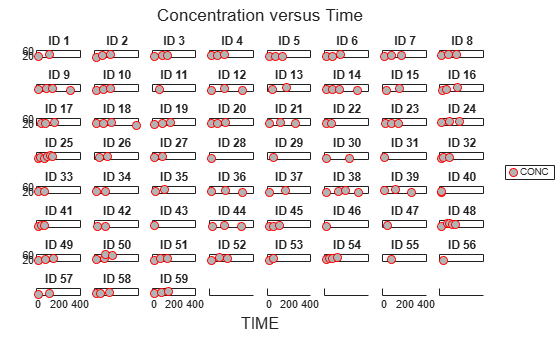Create a One-Compartment PK Model

Create a simple one-compartment PK model, with bolus dose administration and linear clearance elimination, to fit the data.

```pkmd = PKModelDesign; addCompartment(pkmd,'Central','DosingType','Bolus',... 'EliminationType','linear-clearance',... 'HasResponseVariable',true,'HasLag',false); onecomp = pkmd.construct;```

Map model species to response data.

`responseMap = 'Drug_Central = CONC';`

Define Estimated Parameters

The parameters to estimate in this model are the volume of the central compartment (`Central`) and the clearance rate (`Cl_Central`). `sbiofitmixed` calculates fixed and random effects for each parameter. The underlying algorithm computes normally distributed random effects, which might violate constraints for biological parameters that are always positive, such as volume and clearance. Therefore, specify a transform for the estimated parameters so that the transformed parameters follow a normal distribution. The resulting model is

`$log\left({V}_{i}\right)=log\left({\varphi }_{V,i}\right)={\theta }_{V}+{\eta }_{V,i}$`

and

`$log\left(C{l}_{i}\right)=log\left({\varphi }_{Cl,i}\right)={\theta }_{Cl}+{\eta }_{Cl,i},$`

where $\theta$, $eta$, and $\varphi$ are the fixed effects, random effects, and estimated parameter values respectively, calculated for each infant (group) $i$. Some arbitrary initial estimates for V (volume of central compartment) and Cl (clearance rate) are used here in the absence of better empirical data.

`estimatedParams = estimatedInfo({'log(Central)','log(Cl_Central)'},'InitialValue',[1 1]);`

Define Dosing

All infants were given the drug, represented by the `Drug_Central` species, where the dosing schedule varies among infants. The amount of drug is listed in the data variable DOSE. You can automatically generate dose objects from the data and use them during fitting. In this example, `Drug_Central` is the target species that receives the dose.

```sampleDose = sbiodose('sample','TargetName','Drug_Central'); doses = createDoses(data,'DOSE','',sampleDose);```

Fit the Model

Use `sbiofitmixed` to fit the one-compartment model to the data.

`nlmeResults = sbiofitmixed(onecomp,data,responseMap,estimatedParams,doses,'nlmefit');`

Visualize Results

Visualize the fitted results using individual-specific parameter estimates.

`plot(nlmeResults,'ParameterType','individual');`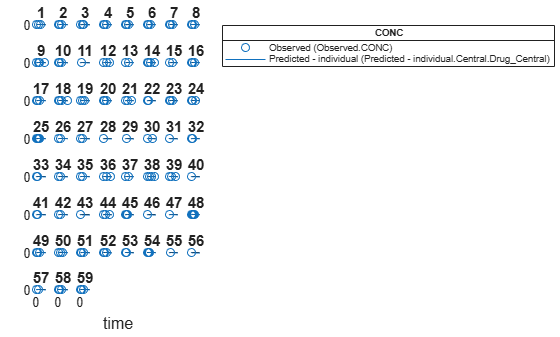Use New Dosing Data to Simulate the Fitted Model

Suppose you want to predict how infants 1 and 2 would have responded under different dosing amounts. You can predict their responses as follows.

Create new dose objects with new dose amounts.

```dose1 = doses(1); dose1.Amount = dose1.Amount*2; dose2 = doses(2); dose2.Amount = dose2.Amount*1.5;```

Use the `predict` function to evaluate the fitted model using the new dosing data. If you want response predictions at particular times, provide the new output time vector. Use the 'ParameterType' option to specify individual or population parameters to use. By default, `predict` uses the population parameters when you specify output times.

```timeVec = [0:25:400]; newResults = predict(nlmeResults,timeVec,[dose1;dose2],'ParameterType','population');```

Visualize the predicted responses while overlapping the experimental data for infants 1 and 2.

```figure; subplot(2,1,1) plot(data.TIME(data.ID == 1),data.CONC(data.ID == 1),'bo') hold on plot(newResults(1).Time,newResults(1).Data,'b') hold off ylabel('Concentration') legend('Observation(CONC)','Prediction') subplot(2,1,2) plot(data.TIME(data.ID == 2),data.CONC(data.ID == 2),'rx') hold on plot(newResults(2).Time,newResults(2).Data,'r') hold off legend('Observation(CONC)','Prediction') ylabel('Concentration') xlabel('Time')```Create a Covariate Model for the Covariate Dependencies

Suppose there is a correlation between volume and weight, and possibly volume and APGAR score. Consider the effect of weight by modeling two of these covariate dependencies: the volume of central (`Central`) and the clearance rate (`Cl_Central`) vary with weight. The model becomes

`$log\left({V}_{i}\right)=log\left({\varphi }_{V,i}\right)={\theta }_{V}+{\theta }_{V/weight}*weigh{t}_{i}+{\eta }_{V,i}$`

and

`$log\left(C{l}_{i}\right)=log\left({\varphi }_{Cl,i}\right)={\theta }_{Cl}+{\theta }_{Cl/weight}*weigh{t}_{i}+{\eta }_{Cl,i}$`

Use the `CovariateModel` object to define the covariate dependencies. For details, see Specify a Covariate Model.

```covModel = CovariateModel; covModel.Expression = ({'Central = exp(theta1 + theta2*WEIGHT + eta1)',... 'Cl_Central = exp(theta3 + theta4*WEIGHT + eta2)'});```

Use `constructDefaultInitialEstimate` to create an `initialEstimates` struct.

`initialEstimates = covModel.constructDefaultFixedEffectValues;`

Use the `FixedEffectNames` property to display the thetas (fixed effects) defined in the model.

`covModel.FixedEffectNames`
```ans = 4x1 cell {'theta1'} {'theta3'} {'theta2'} {'theta4'} ```

Use the `FixedEffectDescription` property to show the descriptions of corresponding fixed effects (thetas) used in the covariate expression. For example, `theta2` is the fixed effect for the weight covariate that correlates with the volume (`Central`), denoted as 'Central/WEIGHT'.

`disp('Fixed Effects Description:');`
```Fixed Effects Description: ```
`disp(covModel.FixedEffectDescription);`
``` {'Central' } {'Cl_Central' } {'Central/WEIGHT' } {'Cl_Central/WEIGHT'} ```

Set the initial guesses for the fixed-effect parameter values for `Central` and `Cl_Central` using the values estimated from fitting the base model.

```initialEstimates.theta1 = nlmeResults.FixedEffects.Estimate(1); initialEstimates.theta3 = nlmeResults.FixedEffects.Estimate(2); covModel.FixedEffectValues = initialEstimates;```

Fit the Model

`nlmeResults_cov = sbiofitmixed(onecomp,data,responseMap,covModel,doses,'nlmefit');`

Display Fitted Parameters and Covariances

`disp('Estimated Fixed Effects:');`
```Estimated Fixed Effects: ```
`disp(nlmeResults_cov.FixedEffects);`
``` Name Description Estimate StandardError __________ _____________________ ________ _____________ {'theta1'} {'Central' } -0.45664 0.078933 {'theta3'} {'Cl_Central' } -5.9519 0.1177 {'theta2'} {'Central/WEIGHT' } 0.52948 0.047342 {'theta4'} {'Cl_Central/WEIGHT'} 0.61954 0.071386 ```
`disp('Estimated Covariance Matrix:');`
```Estimated Covariance Matrix: ```
`disp(nlmeResults_cov.RandomEffectCovarianceMatrix);`
``` eta1 eta2 ________ ________ eta1 0.046503 0 eta2 0 0.041609 ```

Visualize Results

Visualize the fitted results using individual-specific parameter estimates.

`plot(nlmeResults_cov,'ParameterType','individual');`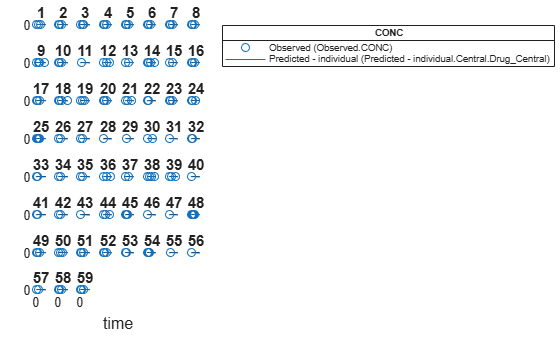Use New Covariate Data to Evaluate the Fitted Model

Suppose you want to explore the responses of infants 1 and 2 using different covariate data, namely `WEIGHT`. You can do this by specifying the new `WEIGHT` data. The `ID` variable of the data corresponds to individual infants.

```newData = data(data.ID == 1 | data.ID == 2,:); newData.WEIGHT(newData.ID == 1) = 1.3; newData.WEIGHT(newData.ID == 2) = 1.4;```

Simulate the responses of infants 1 and 2 using the new covariate data.

`[newResults_cov, newEstimates] = predict(nlmeResults_cov,newData,[dose1;dose2]);`

`newEstimates` contains the updated parameter estimates for each individual (infants 1 and 2) after the model is reevaluated using the new covariate data.

`newEstimates`
```newEstimates=4×3 table Group Name Estimate _____ ______________ _________ 1 {'Central' } 2.5596 1 {'Cl_Central'} 0.0065965 2 {'Central' } 1.7123 2 {'Cl_Central'} 0.0064806 ```

Compare to the estimated values from the original fit using the old covariate data.

```nlmeResults_cov.IndividualParameterEstimates( ... nlmeResults_cov.IndividualParameterEstimates.Group == '1' | ... nlmeResults_cov.IndividualParameterEstimates.Group == '2',:)```
```ans=4×3 table Group Name Estimate _____ ______________ _________ 1 {'Central' } 2.6988 1 {'Cl_Central'} 0.0070181 2 {'Central' } 1.8054 2 {'Cl_Central'} 0.0068948 ```

Visualize the new simulation results together with the experimental data for infant 1 and 2.

```figure; subplot(2,1,1); plot(data.TIME(data.ID == 1),data.CONC(data.ID == 1),'bo') hold on plot(newResults_cov(1).Time,newResults_cov(1).Data,'b') hold off ylabel('Concentration') legend('Observation(CONC)','Prediction','Location','NorthEastOutside') subplot(2,1,2) plot(data.TIME(data.ID == 2),data.CONC(data.ID == 2),'rx') hold on plot(newResults_cov(2).Time,newResults_cov(2).Data,'r') hold off legend('Observation(CONC)','Prediction','Location','NorthEastOutside') ylabel('Concentration') xlabel('Time')```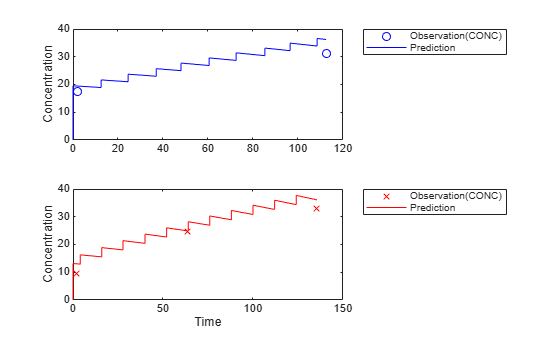References

 Grasela, T. H. Jr., and S. M. Donn. "Neonatal population pharmacokinetics of phenobarbital derived from routine clinical data." Dev Pharmacol Ther 1985:8(6). 374-83.

This example uses data collected on 59 preterm infants given phenobarbital during the first 16 days after birth. Each infant received an initial dose followed by one or more sustaining doses by intravenous bolus administration. A total of between 1 and 6 concentration measurements were obtained from each infant at times other than dose times, for a total of 155 measurements. Infant weights and APGAR scores (a measure of newborn health) were also recorded. Data was described in , a study funded by the NIH/NIBIB grant P41-EB01975.

`load pheno.mat ds`

Visualize the data.

```t = sbiotrellis(ds,'ID','TIME','CONC','marker','o','markerfacecolor',[.7 .7 .7],'markeredgecolor','r','linestyle','none'); t.plottitle = 'States versus Time';```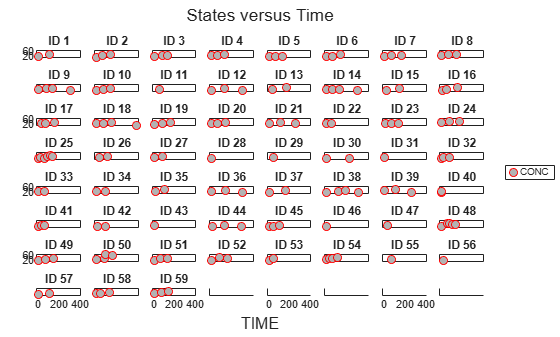Create a one-compartment PK model with bolus dosing and linear clearance to model such data.

```pkmd = PKModelDesign; pkmd.addCompartment('Central','DosingType','Bolus','EliminationType','linear-clearance',... 'HasResponseVariable',true,'HasLag',false); onecomp = pkmd.construct;```

Suppose there is a correlation between the volume of the central compartment (`Central`) and the weight of infants. You can define this parameter-covariate relationship using a covariate model that can be described as

$\mathrm{log}\left({V}_{i}\right)={\theta }_{V}+{\theta }_{V}{WEIGHT}}\ast WEIGH{T}_{i}+{\eta }_{V,i}$,

where, for each ith infant, `V` is the volume, θs (thetas) are fixed effects, η (eta) represents random effects, and `WEIGHT` is the covariate.

```covM = CovariateModel; covM.Expression = {'Central = exp(theta1+theta2*WEIGHT+eta1)'};```

Define the fixed and random effects. The column names of each table must have the names of fixed effects and random effects, respectively.

```thetas = table(1.4,0.05,'VariableNames',{'theta1','theta2'}); eta1 = table(0.2,'VariableNames',{'eta1'});```

Change the group label ID to GROUP as required by the `sbiosampleparameters` function.

`ds.Properties.VariableNames{'ID'} = 'GROUP';`

Generate parameter values for the volumes of central compartments Central based on the covariate model for all infants in the data set.

`phi = sbiosampleparameters(covM.Expression,thetas,eta1,ds);`

You can then simulate the model using the sampled parameter values. For convenience, use the function-like interface provided by a SimFunction object.

First, construct a SimFunction object using the createSimFunction method, specifying the volume (Central) as the parameter, and the drug concentration in the compartment (Drug_Central) as the output of the SimFunction object, and the dosed species.

`f = createSimFunction(onecomp,covM.ParameterNames,'Drug_Central','Drug_Central');`

The data set ds contains dosing information for each infant, and the groupedData object provides a convenient way to extract such dosing information. Convert ds to a groupedData object and extract dosing information.

```grpData = groupedData(ds); doses = createDoses(grpData,'DOSE');```

Simulate the model using the sampled parameter values from phi and the extracted dosing information of each infant, and plot the results. The ith run uses the ith parameter value in phi and dosing information of the ith infant.

```t = sbiotrellis(f(phi,200,doses.getTable),[],'TIME','Drug_Central'); % Resize the figure. t.hFig.Position(:) = [100 100 1280 800];```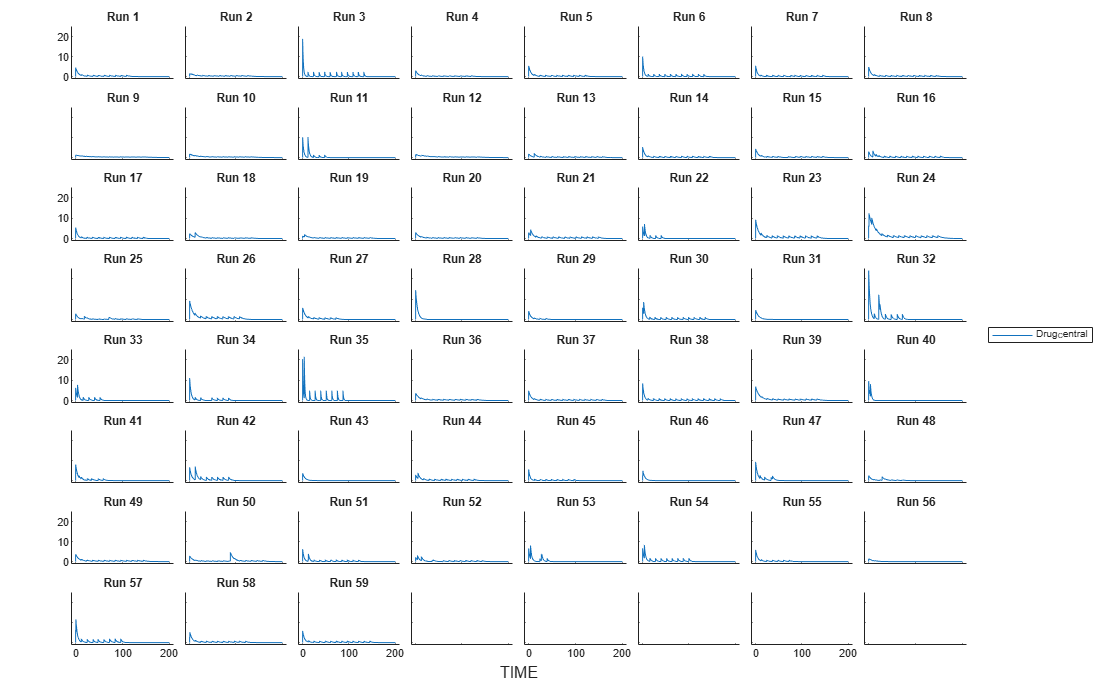## Version History

Introduced in R2011b